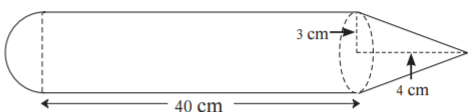# In figure 7.11, a toy made from a hemisphere, a cylinder and a cone is shown. Find the total area of the toy

In figure 7.11, a toy made from a hemisphere, a cylinder and a cone is shown. Find the total area of the toyverified

Radius of the sphere = Radius of the cylinder = Radius of cone = r = 3 cm
Height of the cone, h = 4 cm
Height of the cylinder, H = 40 cm
Let the slant height of the cone be l cm.

$$\therefore l = \sqrt{r^2 + h^2} = \sqrt{3^2 + 4^2} = \sqrt{9 + 16} = \sqrt{25} = 5 cm$$

Total area of the toy

= Curved surface area of hemisphere + Curved surface area of cylinder + Curved surface area of cone

$$= 2\pi r^2 + 2 \pi H + \pi l$$
$$= 2\pi \times \left( 3 \right)^2 + 2\pi \times 3 \times 40 + \pi \times 3 \times 5$$
$$= 18\pi + 240\pi + 15\pi$$
$$= 273\pi {cm}^2$$

Thus, the total area of the toy is 273$$\pi$$ cm2